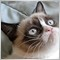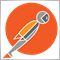# Is it possible to use "function arrays"?963

Is it possible to use function arrays in mql4? As in an array that holds a function in every index so that the following 2 functions can be placed inside an array:

```int SellStopsTotalTF0()
{
int SellStopsTotalTF0=0;
for(int x=0;x<OrdersTotal();x++)
{
{
SellStopsTotalTF0=SellStopsTotalTF0+1;
}
}
return(SellStopsTotalTF0);
}

int SellStopsTotalTF1()
{
int SellStopsTotalTF1=0;
for(int x=0;x<OrdersTotal();x++)
{
{
SellStopsTotalTF1=SellStopsTotalTF1+1;
}
}
return(SellStopsTotalTF1);
}```

```int SellStopsTotalTF[x]()
{
int SellStopsTotalTF[x]=0;
for(int x=0;x<OrdersTotal();x++)
{
{
SellStopsTotalTF[x]=SellStopsTotalTF[x]+1;
}
}
return(SellStopsTotalTF[x]);
}```

Would also need to be able to place a defined array's values (the TimeFrame[x] array's values) inside the order select section as shown above.

Is this or an equivalent at all possible? Any ideas as to how to accomplish this? So its actually 2 questions here:

1. Can we use functions as array values in any way at all?

2. Can we insert an array's index (actual value) as a variable for a function used inside a function array or in any way at all?

Tried starting the EA with

```   for(int x=0;x<ArraySize(TimeFrame);x++)
{
int SellStopsTotal[x]()
{
int SellStopsTotal[x]=0;
for(int x=0;x<OrdersTotal();x++)
{
{
SellStopsTotal[x]=SellStopsTotal[x]+1;
}
}
return(SellStopsTotal[x]);
}
}```

When trying to compile returns that [x] is an invalid array index and that SellStopsTotal function can only be declared globally; both things which imply that what I'm trying to do is just not supported.21724

1. You can't create an array of functions. Create a helper function:
```int SellStopsTotal(int iWhich){
switch(iWhich){
case 0: return SellStopsTotalTF0();
case 1: return SellStopsTotalTF1();
}
Print(...); return EMPTY;
}
```

2. The only thing different between TF0() and TF1() is the timeframe. Instead, pass it as an argument.
```int SellStopsTotalTF(ENUM_TIMEFRAMES tf=PERIOD_CURRENT)
{
if(tf == PERIOD_CURRENT)  tf = (ENUM_TIMEFRAMES)_Period;
int SellStopsTotal=0;
for(int x=0;x<OrdersTotal();x++)
{
{
++SellStopsTotal;
}
}
return SellStopsTotal;
}```

3. `OrderType()==OP_SELLSTOP && _Period==TimeFrame[x])`
An order does not have a timeframe. Period is the current chart's. That is bogus. If you really want to find orders per TF, open them with a magic number MNbase+TF and then you can filter by MN.1189

Is it possible to use function arrays in mql4? ...

Well, you could have an array of class instances which feature some function. Like so:

```class Agent {
public:
};

class AgentMulder : public Agent {
public:
// cool stuff going on
}
};

class AgentScully : public Agent {
public:
// other stuff going on
}
};

Agent *SpecialAgents;

int OnInit() {
SpecialAgents=new AgentMulder;
SpecialAgents=new AgentScully;
}
```

Albeit that @whroeder1 is spot on with this advice, there's no need to do it that complex.2619

FWIW, you actually can have an array of function pointers in MQL. The functions need to have the same return types and params, but it can be done.

```void p1() { Print("func 1"); }
void p2() { Print("func 2"); }

void OnStart()
{
typedef void (*TFunc)(void);
TFunc funcs;
funcs = p1;
funcs = p2;
for (int i=0; i<ArraySize(funcs); i++) {
funcs[i]();
}
}```

That being said, I've never had a real-life practical use-case for this pattern in MQL.963

whroeder1:
1. You can't create an array of functions. Create a helper function:

2. The only thing different between TF0() and TF1() is the timeframe. Instead, pass it as an argument.

3. An order does not have a timeframe. Period is the current chart's. That is bogus. If you really want to find orders per TF, open them with a magic number MNbase+TF and then you can filter by MN.

Thanks I got it sorted out963

nicholi shen:

FWIW, you actually can have an array of function pointers in MQL. The functions need to have the same return types and params, but it can be done.

That being said, I've never had a real-life practical use-case for this pattern in MQL.

Great to know963

lippmaje:

Well, you could have an array of class instances which feature some function. Like so:

Albeit that @whroeder1 is spot on with this advice, there's no need to do it that complex.

Excellent!!!